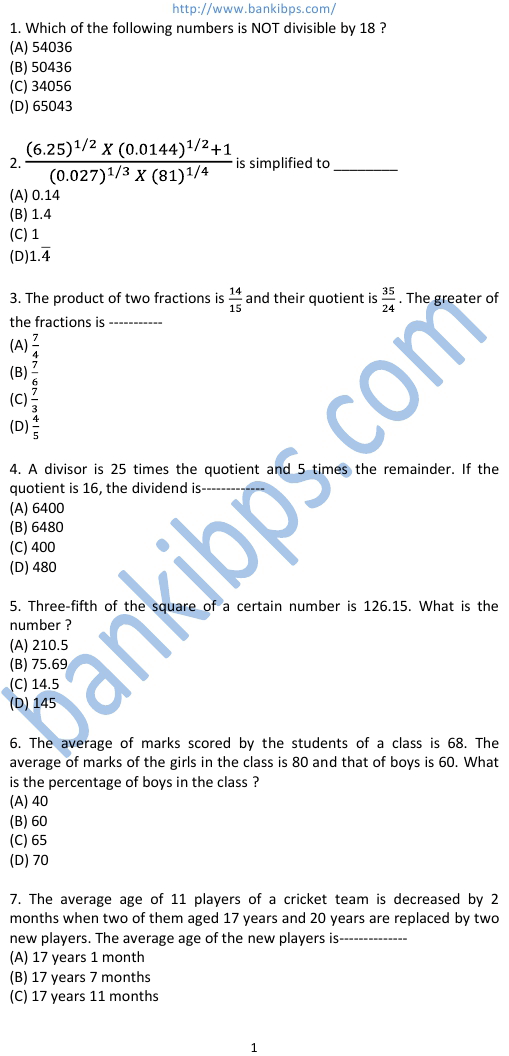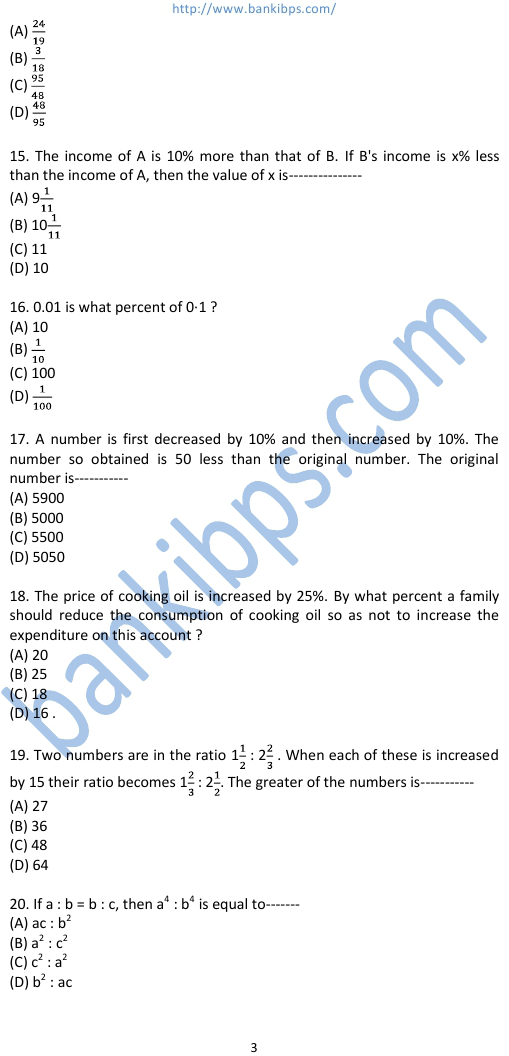# Previous Question Paper of SSC Combined Graduate Level Exams

Three-fifth of the square of a certain number is 126.15. What is the number The average of marks scored by the students of a class is 68. The average of marks of the girls in the class is 80 and that of boys is 60. What is the percentage of boys in the The average age of 11 players of a cricket team is decreased by 2 months when two of them aged 17 years and 20 years are replaced by two new players. The average age Out of three numbers the first is twice the second and is half of the third. If the average of the three numbers is 56. then difference of first and third numbers The average monthly salary of previous question paper of ssc combined graduate level exams the workers in a workshop is Rs. 8500. If the average monthly salary of 7 technicians is Rs. 10,000 and average monthly salary The average of marks of 28 students in Mathematics was 50; 8 students left the school. then this average increased by 5. What is the average of marks obtained by the students In a class, the number of girls is 20% more than that of the boys. The strength of the class is 66. If 4 more girls are admitted to the class. the ratio of the number of boys to that The price of cooking oil is increased by 25%. By what percent a family should reduce the consumption of cooking oil so as not to increase the expenditure on this.